Next: Example 6.4: Energy stored Up: Capacitance Previous: Example 6.2: Dielectric filled

## Example 6.3: Equivalent capacitance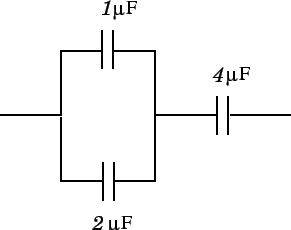Question: A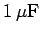and a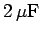capacitor are connected in parallel, and this pair of capacitors is then connected in series with a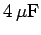capacitor, as shown in the diagram. What is the equivalent capacitance of the whole combination? What is the charge on thecapacitor if the whole combination is connected across the terminals of a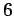V battery? Likewise, what are the charges on theandcapacitors?

Answer: The equivalent capacitance of theandcapacitors connected in parallel is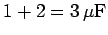. When a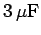capacitor is combined in series with acapacitor, the equivalent capacitance of the whole combination is given by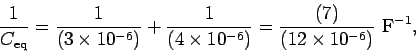and so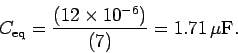The charge delivered by theV battery is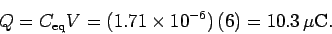This is the charge on thecapacitor, since one of the terminals of the battery is connected directly to one of the plates of this capacitor.

The voltage drop across thecapacitor is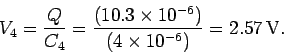Thus, the voltage drop across theandcombination must be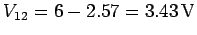. The charge stored on theis given by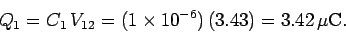Likewise, the charge stored on thecapacitor is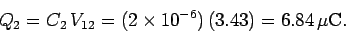Note that the total charge stored on theandcombination is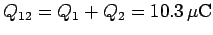, which is the same as the charge stored on thecapacitor. This makes sense because theandcombination and thecapacitor are connected in series.Next: Example 6.4: Energy stored Up: Capacitance Previous: Example 6.2: Dielectric filled
Richard Fitzpatrick 2007-07-14